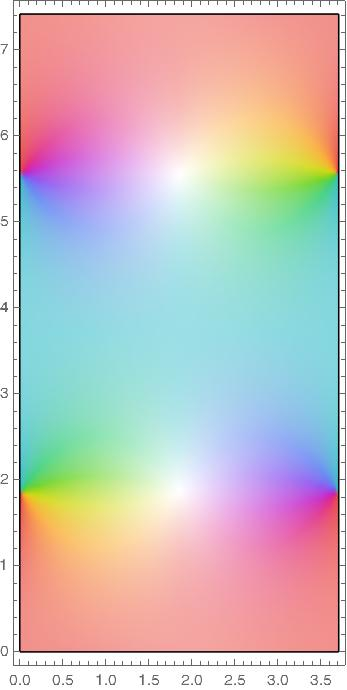# Fundamental rectangles for Jacobi elliptic functions

Elliptic functions are doubly periodic and so their values everywhere in the complex plane are determined by their values in a fundamental parallelogram. We will assume the parameter m is real and between 0 and 1 so that the fundamental parallelograms are rectangles aligned with the real and imaginary axes.

The functions sn, ns, cd, and dc all have a fundamental rectangle with vertices at o and 4K(m) + 2K(1-m) i.

The functions cn, nc, ds, and sd all have a fundamental rectangle with vertices at o and 4K(m) + 4K(1-m) i.

The functions cs, sc, dn, and nd all have a fundamental rectangle with vertices at o and 2K(m) + 4K(1-m) i.

In the plots below m = 1/2 so that m = 1 – m.

Pinwheels of color indicate zeros and white areas indicate poles.

Note that the reciprocal of a function swaps the letters in the name, e.g. ns(z) = 1/sn(z). Taking the reciprocal swaps zeros and poles.

## snThis function was plotted in Mathematica using

```    K = EllipticK[1/2]
ComplexPlot[JacobiSN[z, 1/2],
{z, 0, 4 K + 2 I K},
AspectRatio -> Automatic]
```

and the rest of the plots were made analogously.

## ns## cd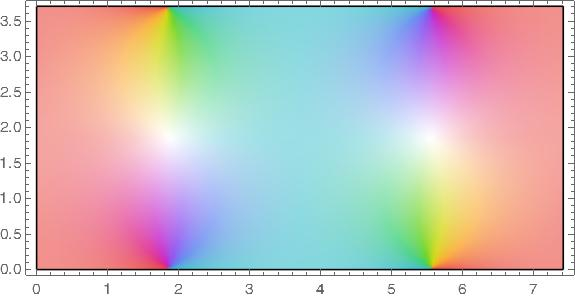## dc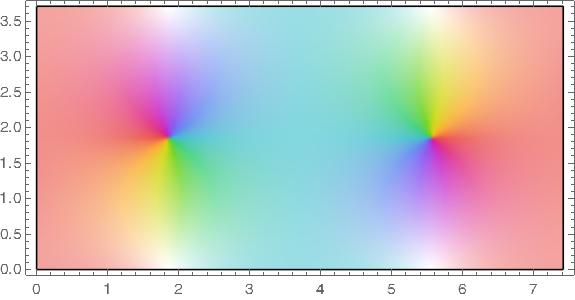## cn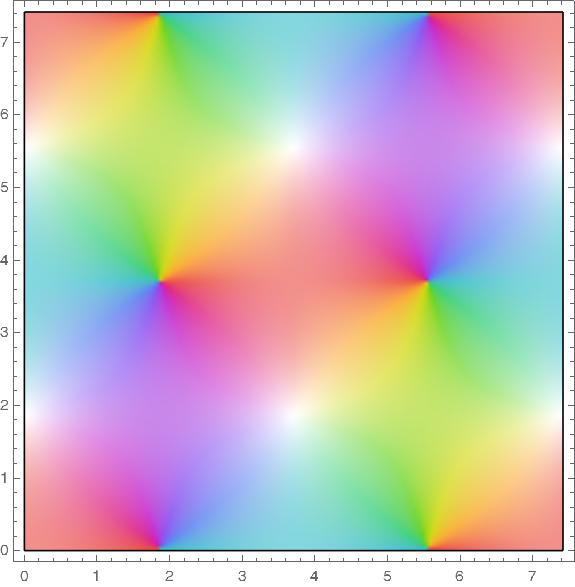## nc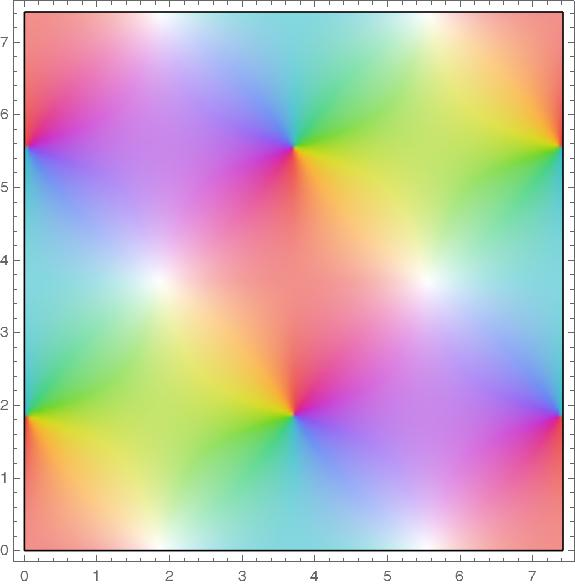## ds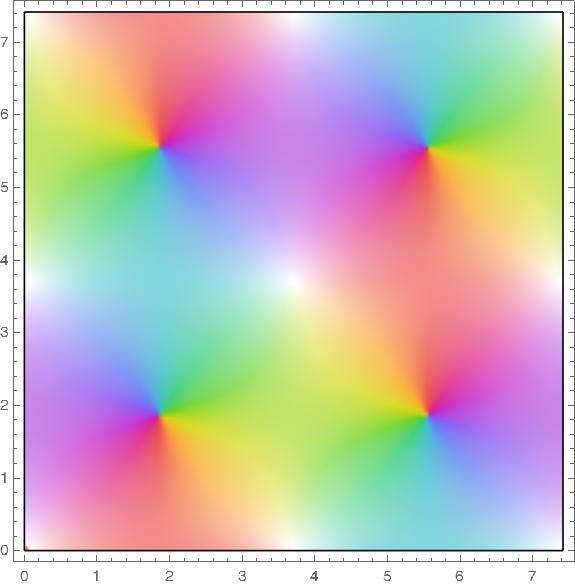## sd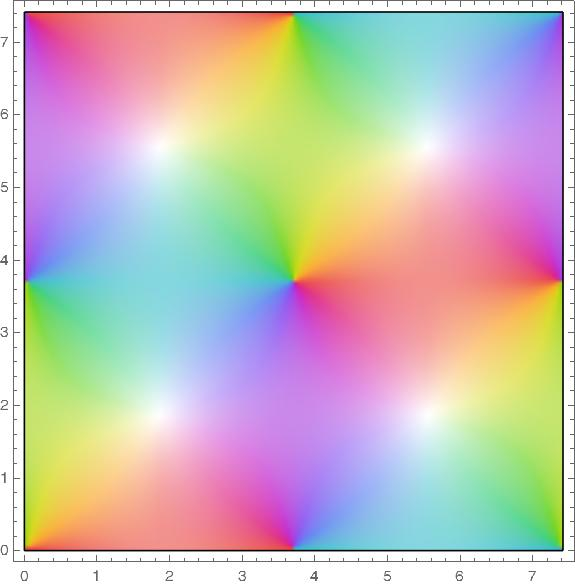## cs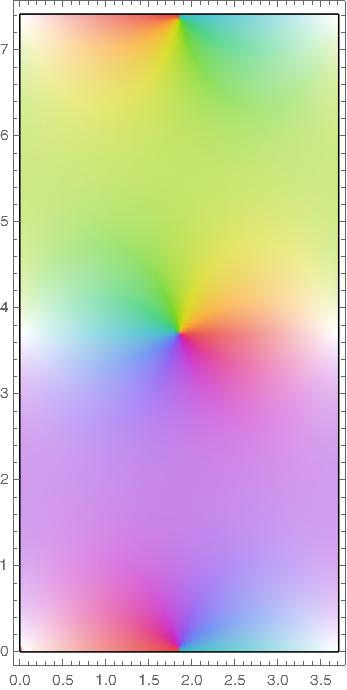## sc## dn## nd